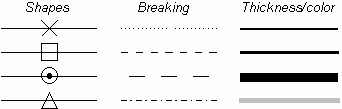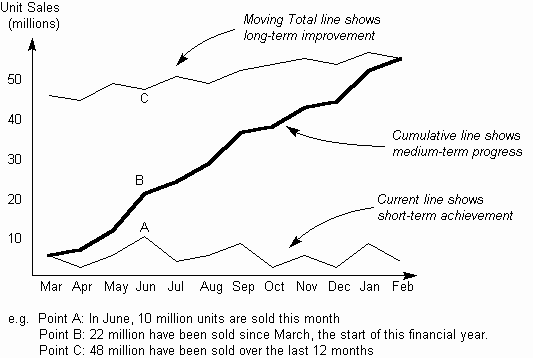The Psychology of Quality and More

# Line Graph: Practical variations

The Quality Toolbook > Line Graph > Practical variations

## Practical variations

• Use point markers to emphasize the value at each point. Omit them to emphasize the overall shape of the graph.
• There are a number of common ways of showing different point markers and lines to enable them to be distinguished (see the illustration). If available, color is a very good differentiator.Fig. 1. Differentiating lines

• Where a line varies up and down a lot, a simple way of drawing a trend line is a 3-point average. In this, for each point, a new point is plotted which is the average of the previous, the current and the next point.
• In an Area Graph, the area beneath the line is emphasized by filling it in (often in color). This can be used as a variation on a Stacked Bar Chart.
• A Z-Chart plots on one chart the current, cumulative and moving total figures for any measured value across time, as in The illustration. It is useful for showing progress in both the short and longer term. The current line shows the variation each period, whilst the moving total smooths out any seasonal variations, showing longer term progress. The cumulative line joins these and may show progress towards a goal.Fig. 2. Z-Chart

### You can buy books here

And the big
paperback book